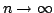Statistica Sinica 18(2008), 1025-1045

AN ALTERNATIVE VIEW OF

THE DECONVOLUTION PROBLEM

Aurore Delaigle

University of Bristol and University of Melbourne

Abstract: The deconvolution kernel density estimator is a popular technique for solving the deconvolution problem, where the goal is to estimate a density from a sample of contaminated observations. Although this estimator is optimal, it suffers from two major drawbacks: it converges at very slow rates (inherent to the deconvolution problem) and can only be calculated when the density of the errors is completely known. These properties, however, follow from a classical asymptotic view of the problem which lets the sample sizebut where the error varianceis supposed to be fixed. We argue that, in many situations, a more appropriate way to derive asymptotic properties for the deconvolution problem is to consider that bothand. In this context, not only do the rates of convergence of the deconvolution kernel density estimator improve considerably, but it is also possible to consistently estimate the target density with only little knowledge of the error density. In particular, the deconvolution kernel density estimator becomes robust against error misspecification and a low-order approximation developed in the literature becomes consistent. We propose a data-driven procedure for the low-order method and investigate the numerical performance of the various estimators on simulated and real data examples.

Key words and phrases: Asymptotic results, bandwidth selection, classical errors, kernel method, measurement errors, smoothing.